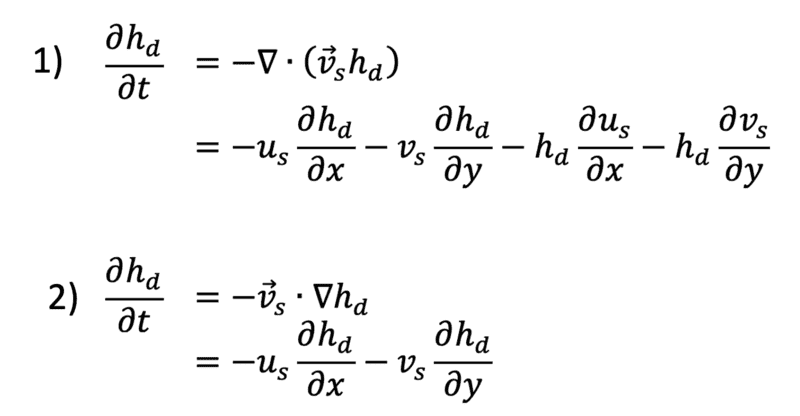# How can I interpret the 2D advection equation?

• A
• jones1234

#### jones1234

TL;DR Summary
How can I interpret the 2D advection equation?
I want to model the advection of debris rock layer with a thickness hd on top of a glacier through ice flow with velocity components u and v. Can anybody explain the physical difference between these 2 equations and which one I should take? Thanks•Delta2

Please provide a diagram. Are the v's in the equations the averages with respect to z over the thickness of the layer or the values at the top of the layer?

Please provide a diagram. Are the v's in the equations the averages with respect to z over the thickness of the layer or the values at the top of the layer?
u_s = the x-component of the surface velocity,
v_s = the y-component of the surface velocity,
h_d = the thickness of the rock layer

u_s = the x-component of the surface velocity,
v_s = the y-component of the surface velocity,
h_d = the thickness of the rock layer
Is the rock moving as a rigid layer or is it deforming?

Is the rock moving as a rigid layer or is it deforming?
The rocks are not deforming. They are on top of the ice and get transported by the ice.

The rocks are not deforming. They are on top of the ice and get transported by the ice.
Then u and v do not vary with x and y

Then u and v do not vary with x and y
The most important part of modeling is actually understanding the physics of the process and then deriving the underlying model. Do you see how eq. (2) can be obtained from eq. (1) with the comments of @Chestermiller ?

If you haven't done so already, it will also be good for your understanding to go back even one step further and ask yourself why the change of debris height in time is given by this divergence term in the first place. The diagram that @Chestermiller asked for will be crucial for this understanding

•Delta2